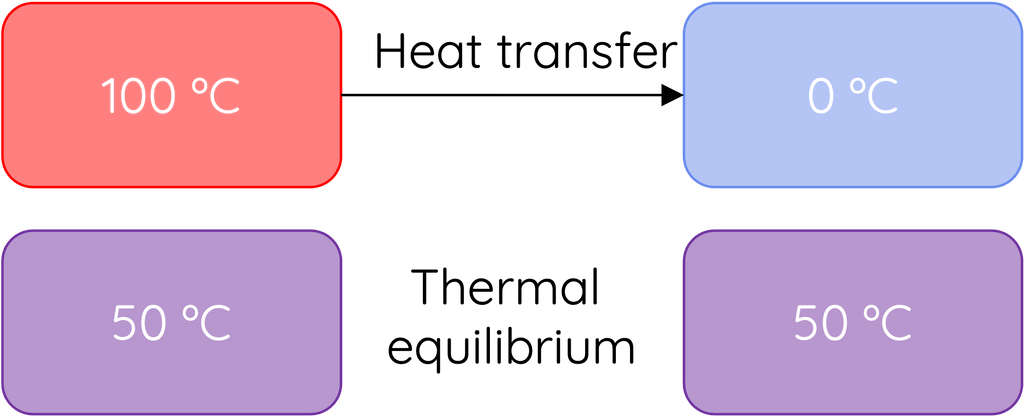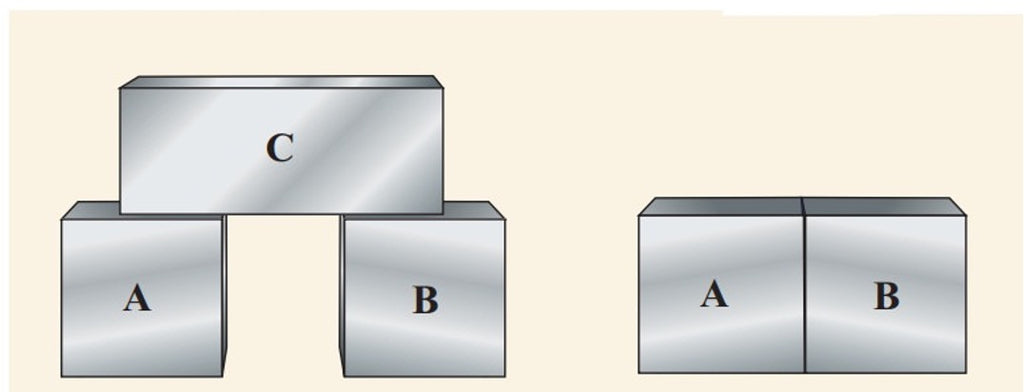# Thermal Equilibrium and Energy Transfer

This topic is part of the HSC Physics course under the section Thermodynamics.

### HSC Physics Syllabus

• explain the concept of thermal equilibrium (ACSPH022)
• investigate energy transfer by the process of:
– conduction
– convection

When we talk about energy transfer in physics, especially in the context of temperature and heat, there are three primary mechanisms to consider: conduction, convection, and radiation. Understanding these processes, as well as the concept of thermal equilibrium, is essential for grasping how energy moves and how objects reach the same temperature.

### Thermal Equilibrium

Thermal equilibrium is achieved when two systems in physical contact with each other no longer exchange any heat, meaning they have the same temperature. It's essential to understand that this does not necessarily mean the systems have the same amount of internal energy, just that their temperatures are equal.If System A is at a higher temperature than System B and they are brought into contact, heat will flow from A (the hotter system) to B (the cooler system) until they both reach the same temperature. Once this happens, they are in thermal equilibrium, and no more heat exchange occurs.

It is important to understand that the final temperature shared by the substances at thermal equilibrium is not always the average of their initial temperatures (shown in the diagram above). Whether this occurs depends on the mass of the substances and their specific heat capacities. Two substances will only reach a temperature that is the average of their starting temperatures if they have equal mass and specific heat capacities.

Imagine a hot cup of tea placed in a cooler room. Over time, the tea will cool down, and the room might become slightly warmer. This exchange of heat will continue until the temperature of the tea matches the room's temperature. At this point, they have reached thermal equilibrium. No more heat energy is transferred between the tea and its surroundings.

### Zeroth Law of Thermodynamics

The Zeroth Law of Thermodynamics states that if two systems are each in thermal equilibrium with a third system, then they are in thermal equilibrium with each other.In simpler terms, if System A is in thermal equilibrium with System C, and System B is also in thermal equilibrium with System C, then System A and System B must be in thermal equilibrium with each other.

This law essentially establishes the concept of temperature. It is the foundation upon which the notion of temperature scale is built, enabling us to measure and compare the temperature of different objects. The name "zeroth law" was assigned because after the first, second, and third laws of thermodynamics were already established, it was realised that this fundamental postulate should precede the other laws, and thus was named the "zeroth" law.

### Example 1 – Thermal Equilibrium Between Same Substances

Two identical metal blocks, Block A and Block B have initial temperatures of 30ºC and 50ºC respectively. Both blocks are placed in contact within an isolated system. What will be their final temperature when thermal equilibrium is reached? Assume the masses and specific heat capacities of both blocks are the same.

Solution:

When two objects of different temperatures come into contact, the hotter object will lose heat to the cooler object until they both have the same temperature. The amount of heat lost by the hotter object will be equal to the amount of heat gained by the cooler object.

$$\Delta T_A = T - 30 \\$$

$$\Delta T_B = 50 - T$$

Using the heat equation:

$$Q = mc\Delta T \\$$

Heat gained by Block A = Heat lost by Block B:

$$m \times c \times \Delta T_A = m \times c \times (-\Delta T_B)$$

Substituting the values:

$$m \times c \times (T - 30) = m \times c \times (50 - T)$$

Solving for T:

$$T - 30 = 50 -T$$

$$2T = 80$$

$$T = 40^\circ \text{C}$$

### Example 2 – Thermal Equilibrium Between Different Substances

Suppose you have 0.2 kg of water initially at 20ºC and a 0.3 kg aluminium block initially at 80ºC. The two are placed in contact within an isolated system. After some time, they achieve thermal equilibrium. What is their final temperature?

Use the following information for this question:

• cwater = 4184 J kg–1 K–1
• caluminium = 900 J kg–1 K–1

Solution:

Using the heat equation

$$Q = mc\Delta T$$

Heat gained by the water = Heat lost by the metal:

$$m_{\text{water}} \times c_{\text{water}} \times (T - 20) = m_{\text{metal}} \times c_{\text{metal}} \times (80 - T)$$

Substituting the values and solving for T:

$$0.2 \times 4184 \times (T - 20) = 0.3 \times 900 \times (80 - T)$$

$$T = 34.6^\circ \text{C}$$

### Energy Transfer Mechanisms### 1. Conduction

Conduction is the transfer of thermal energy through a material without the material itself moving. This mode of transfer occurs due to the vibration of atoms and molecules in a substance.In a solid, for example, particles are closely packed together. When one particle vibrates because of an increase in energy (temperature), it bumps into its neighbours, transferring some of its energy to them. This chain reaction continues throughout the material until energy has been spread evenly.

Examples of conduction in everyday life:

• Metal spoons get hot when left in a hot drink.
• Ironing clothes. The heat from the iron plate is conducted to the clothes, making them warm.

The concept of thermal conduction is discussed separately here

### 2. Convection

Convection involves the movement of fluids (liquids and gases). As a fluid becomes hotter, it becomes less dense and rises. Conversely, cooler fluid is denser and sinks.

What is a convection current?

Hotter regions of a fluid rise because they are less dense, and cooler regions sink because they are denser. This cycle creates a convection current which transfers heat from the bottom of a fluid to its top.For example, consider a pot of water being heated on a stove. The water at the bottom of the pot, in direct contact with the heat source, warms up first. As this layer of water heats, it expands slightly and becomes less dense, causing it to rise. As it moves upwards, the cooler water above sinks down to take its place and is subsequently heated. This cyclic process of rising warm water and sinking cooler water creates convection currents that effectively distribute the heat throughout the pot.

Examples of convection in everyday life:

• Boiling water in a pot. The hot water at the bottom rises to the top, and the cooler water at the top sinks to the bottom.
• Sea breezes. During the day, land heats up faster than the sea. The hot air above the land rises, and the cooler air from the sea rushes in to take its place.

Radiation involves the transfer of energy through electromagnetic waves. Unlike conduction and convection, radiation does not require a medium; it can occur in a vacuum.All objects emit electromagnetic radiation in the form of infrared rays. Hotter objects emit more radiation than cooler ones. When these rays strike another object, they increase its energy and, consequently, its temperature.

Examples of radiation in everyday life:

• Feeling warmth from the Sun, even in space where there's a vacuum.
• Feeling the heat from a campfire, even if you are standing far away from it.